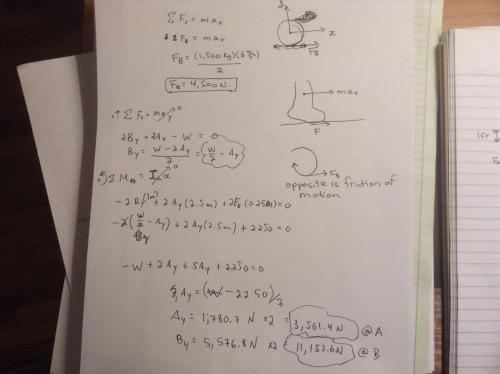## Recommended Posts

Hello just re-did my old quiz from 2014 and for b) I got $F_{B} = 4,500 N, B_{y} = 13,078.9 N, A_{y} = 1,636.1 N$. Did you get these answers as well? Thanks!

##### Share on other sites

Hello just re-did my old quiz from 2014 and for b) I got $F_{B} = 4,500 N, B_{y} = 13,078.9 N, A_{y} = 1,636.1 N$. Did you get these answers as well? Thanks!

Yes. No. No

##### Share on other sites

Yes. No. No

I attempted my calculations again. Still got the same answer for $B_y, A_y$. What'd you get?

##### Share on other sites

I attempted my calculations again. Still got the same answer for $B_y, A_y$. What'd you get?

how many wheels does this car have?

##### Share on other sites

how many wheels does this car have?

Frictionless front wheels and 2 back wheels with $F_b$ applied at each.

##### Share on other sites

Frictionless front wheels and 2 back wheels with $F_b$ applied at each.

I thought that would be enough of a hint. A quote from the question

"and the normal reaction at EACH of the TWO wheels at A and B respectively"

I make that four wheels. Your answer spreads a normal force equalling the force of gravity in the opposite direction over two wheels - one at the front and one at the back

##### Share on other sites

I thought that would be enough of a hint. A quote from the question

"and the normal reaction at EACH of the TWO wheels at A and B respectively"

I make that four wheels. Your answer spreads a normal force equalling the force of gravity in the opposite direction over two wheels - one at the front and one at the back

Yeah that was where I got struck on - the wording of the question. I did my calculations and I hope this is right: $F_B=4,500N, B_y=5,576.8N, and A_y=1,780.7N$. If you want the TOTAL reactions at each A and B then just multiply Ay and By by two.

##### Share on other sites

Yeah that was where I got struck on - the wording of the question. I did my calculations and I hope this is right: $F_B=4,500N, B_y=5,576.8N, and A_y=1,780.7N$. If you want the TOTAL reactions at each A and B then just multiply Ay and By by two.

Looks Good

Looks Good

##### Share on other sites

Yeah that was where I got struck on - the wording of the question. I did my calculations and I hope this is right: $F_B=4,500N, B_y=5,576.8N, and A_y=1,780.7N$. If you want the TOTAL reactions at each A and B then just multiply Ay and By by two.

I don't like it; from a quick glom it looks as if you have torque around the CoM - which is wrong.

##### Share on other sites

I don't like it; from a quick glom it looks as if you have torque around the CoM - which is wrong.

I think it balances with the answers given. Did you include the moment from the thrust?
##### Share on other sites

I think it balances with the answers given. Did you include the moment from the thrust?

Yeah - but I made the same mistake EvoN made - after pointing he was using only one set of wheels I used only one wheels worth of thrust

##### Share on other sites

Yeah - but I made the same mistake EvoN made - after pointing he was using only one set of wheels I used only one wheels worth of thrust

Here's a screenshot of calculations that I did yesterday. I put down 2 Fb for friction force at both back wheels and 2 normal reactions at each A and B. Is it good?##### Share on other sites

Here's a screenshot of calculations that I did yesterday. I put down 2 Fb for friction force at both back wheels and 2 normal reactions at each A and B. Is it good?

If it was a test or assignment it would depend on who is marking it. Just my opinion, but it is better to circle the answers to the questions than double them, even if it somewhat makes sense to do so by adding "at A" and "at B". It would be even more important if a mistake was made and you did not get the right answers.

##### Share on other sites

If it was a test or assignment it would depend on who is marking it. Just my opinion, but it is better to circle the answers to the questions than double them, even if it somewhat makes sense to do so by adding "at A" and "at B". It would be even more important if a mistake was made and you did not get the right answers.

Yeah either way I'm confident with my calculations. I only care about the "process" not the answer. Thanks guys!

## Create an account

Register a new account